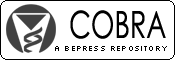## COBRA Preprint Series

#### Abstract

The problems of identifying changes at unknown times and of estimating the location of changes in stochastic processes are referred to as "the change-point problem" or, in the Eastern literature, as "disorder".

The change-point problem, first introduced in the quality control context, has since developed into a fundamental problem in the areas of statistical control theory, stationarity of a stochastic process, estimation of the current position of a time series, testing and estimation of change in the patterns of a regression model, and most recently in the comparison and matching of DNA sequences in microarray data analysis.

Numerous methodological approaches have been implemented in examining change-point models. Maximum-likelihood estimation, Bayesian estimation, isotonic regression, piecewise regression, quasi-likelihood and non-parametric regression are among the methods which have been applied to resolving challenges in change-point problems. Grid-searching approaches have also been used to examine the change-point problem.

Statistical analysis of change-point problems depends on the method of data collection. If the data collection is ongoing until some random time, then the appropriate statistical procedure is called sequential. If, however, a large finite set of data is collected with the purpose of determining if at least one change-point occurred, then this may be referred to as non-sequential. Not surprisingly, both the former and the latter have a rich literature with much of the earlier work focusing on sequential methods inspired by applications in quality control for industrial processes. In the regression literature, the change-point model is also referred to as two- or multiple-phase regression, switching regression, segmented regression, two-stage least squares (Shaban, 1980), or broken-line regression.

The area of the change-point problem has been the subject of intensive research in the past half-century. The subject has evolved considerably and found applications in many different areas. It seems rather impossible to summarize all of the research carried out over the past 50 years on the change-point problem. We have therefore confined ourselves to those articles on change-point problems which pertain to regression.

The important branch of sequential procedures in change-point problems has been left out entirely. We refer the readers to the seminal review papers by Lai (1995, 2001). The so called structural change models, which occupy a considerable portion of the research in the area of change-point, particularly among econometricians, have not been fully considered. We refer the reader to Perron (2005) for an updated review in this area. Articles on change-point in time series are considered only if the methodologies presented in the paper pertain to regression analysis.

#### Disciplines

Statistical Methodology | Statistical Theory

COinS